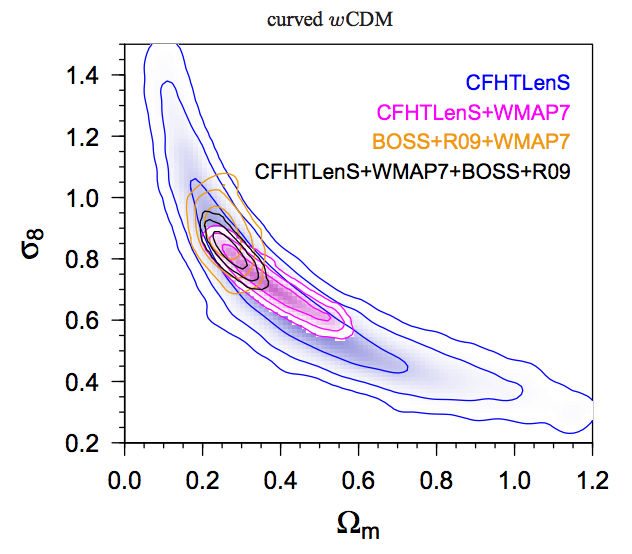# CFHTLenS: Combined probe cosmological model comparison using 2D weak gravitational lensingAuthors: M. Kilbinger, et al. Journal: MNRAS Year: 2013 Download: ADS | arXiv

## Abstract

We present cosmological constraints from 2D weak gravitational lensing by the large-scale structure in the Canada-France Hawaii Telescope Lensing Survey (CFHTLenS) which spans 154 square degrees in five optical bands. Using accurate photometric redshifts and measured shapes for 4.2 million galaxies between redshifts of 0.2 and 1.3, we compute the 2D cosmic shear correlation function over angular scales ranging between 0.8 and 350 arcmin. Using non-linear models of the dark-matter power spectrum, we constrain cosmological parameters by exploring the parameter space with Population Monte Carlo sampling. The best constraints from lensing alone are obtained for the small-scale density-fluctuations amplitude sigma_8 scaled with the total matter density Omega_m. For a flat LambdaCDM model we obtain sigma_8(Omega_m/0.27)^0.6 = 0.79+-0.03.
We combine the CFHTLenS data with WMAP7, BOSS and an HST distance-ladder prior on the Hubble constant to get joint constraints. For a flat LambdaCDM model, we find Omega_m = 0.283+-0.010 and sigma_8 = 0.813+-0.014. In the case of a curved wCDM universe, we obtain Omega_m = 0.27+-0.03, sigma_8 = 0.83+-0.04, w_0 = -1.10+-0.15 and Omega_K = 0.006+0.006-0.004.
We calculate the Bayesian evidence to compare flat and curved LambdaCDM and dark-energy CDM models. From the combination of all four probes, we find models with curvature to be at moderately disfavoured with respect to the flat case. A simple dark-energy model is indistinguishable from LambdaCDM. Our results therefore do not necessitate any deviations from the standard cosmological model.